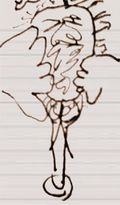# ℱourier Trans∱ormYou just woke up, your mind is hazy. Time to perform a Fourier Transform.

In Theometry, the Fourier Transform is a method of decomposing the life of an individual into a linear combination of sins and cos's (pronounced causes). Pope Jean-Baptiste Joseph Fourier XII invented the transform as a method of efficiently passing information to God for use during the Final Judgment.

The sins of a person's life are passed to priests discretely, during confession. However, as God wishes to evaluate sins over an entire lifetime, and therefore continuously over an interval, discrete sampling of Sins is not sufficient to determine the fate of an individual.

When priests refer to the Inverse Fourier Transform being done at the Pearly Gates to evaluate a person's life, they refer to St. Peter's Inversion Formula, which recompiles the data collected across an individual's entire lifetime. The following formula is effective, but fairly expensive for mortals to compute, requiring the services of several cardinals on several Sunday Masses. (God, of course, has the Earth at his disposal.)

<latex>f(t) = \mathcal{F}^{-1}(F)(t) = \frac{1}{\sqrt{2\pi}} \int_{-\infty}^\infty F(\omega) e^{i\omega t}\,d\omega.</latex>

In the prior equation, <latex>\omega</latex> is determined at the baptism and stored until required for processing later on.

In recent years, however, the Vatican has suggested the adoption of Discrete Fourier Transforms, which require fewer church services to compute. The length of funerals is thus cut in half, greatly reducing the length of a service.

<latex>x_k = \frac{1}{n} \sum_{j=0}^{n-1} f_j e^{2\pi ijk/n} \quad \quad k = 0, ... ,n-1</latex>

There is a remarkable theorem, due to Bishop Karl Ludwig Wierestrass, that makes possible the calculation of Fourier Transforms using Gregorian Chants. However, its practical application has been never accomplished, because of mysterious reasons.

For those without comedic tastes, the self-proclaimed experts at Wikipedia have an article about Fourier transform.